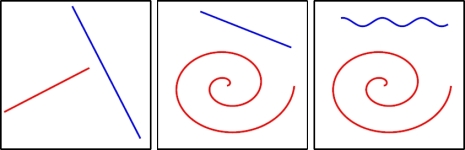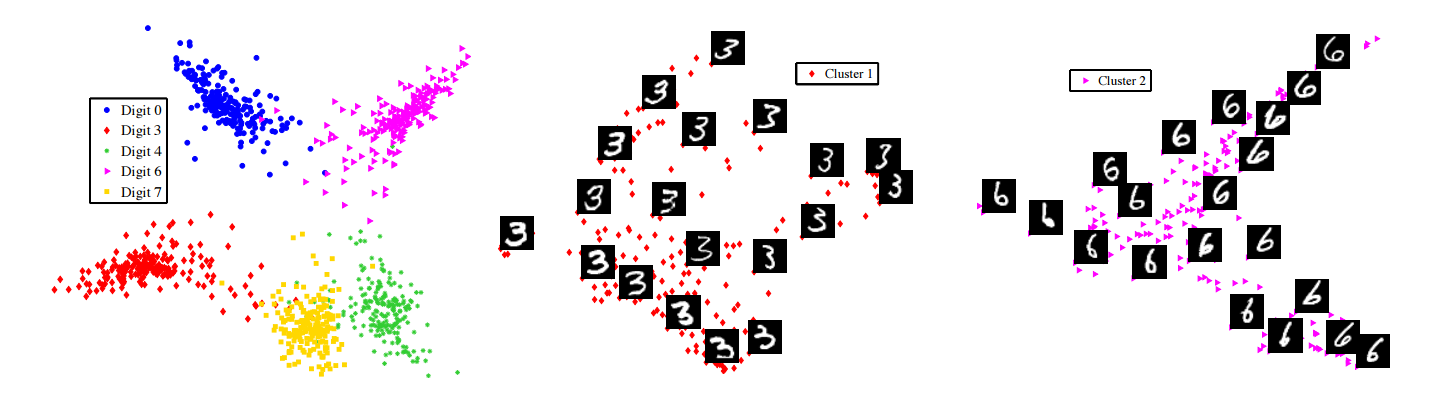Manifold Clustering
Project Summary
Nonlinear dimensionality reduction refers to the problem of finding a low dimensional representation for a set of points lying on a nonlinear manifold embedded in a high dimensional space. This problem is fundamental to many problems in computer vision, machine learning and pattern recognition, because most datasets often have fewer degrees of freedom than the dimension of the data. In computer vision, for example, the number of pixels in an image can be rather large, yet most computer vision models use only a few parameters to describe the geometry, photometry and dynamics of the scene. The question of how to detect and represent low dimensional structure in high dimensional data is fundamental to many disciplines and several attempts had been made in the past in different areas to address this.

Over the past few years, various techniques have been developed for learning a low dimensional representation of a nonlinear manifold embedded in a high dimensional space. Although the goals of dimensionality reduction, classification and segmentation have always been intertwined with each other, considerably less work has been done on extending nonlinear dimensionality reduction techniques for the purposes of clustering data living on different manifolds. Unfortunately, most of these techniques are limited to the analysis of a single connected nonlinear manifold and suffer from degeneracies when applied to linear manifolds (subspaces). Figure 1 shows the types of manifolds to be segmentated into different groups.Figure 1: Types of manifolds to be clustered.
locally linear manifold clustering
We address the problem of simultaneous nonlinear dimensionality reduction and clustering of data points drawn from multiple linear and nonlinear manifolds. We focus our investigation on the LLE algorithm, which compute a low-dimensional embedding from the eigenvectors of a matrix M that depends on local properties of the data. We show that if the data lives in a disconnected union of m connected manifolds, of which n &le m are subspaces of dimensions di, i=1...n, then the null space of M contains m eigenvectors that give the segmentation of the data and &sumi=1...n di eigenvectors that give the embedding coordinates for the subspaces. Therefore, in the case of nonlinear manifolds (n=0) the segmentation of the data can be obtained directly by looking at the null space of M. In the case of subspaces (n=m), contrary to intuition the situation is more difficult, because the nullspace of M contains both segmentation and embedding eigenvectors. We resolve this difficulty by showing that the variance of the segmentation eigenvectors is always smaller than that of the embedding eigenvectors. This leads to a new algorithm for clustering both linear and nonlinear manifolds by solving a generalized eigenvalue problem.

Application to Motion Segmentation
We apply our algorithm to the problem of segmenting multiple rigid-body motions in video. Given N point trajectories in F frames of a video sequence, the goal is to segment these trajectories according to m rigid motions. Depending on the camera projection models, these point trajectories lies on different manifolds. For affine cameras, there are m subspaces of dimensions 2, 3, or 4. For perspective cameras, there are m multilinear manifolds of dimension 3. Experiments on several sequences demonstrate that our algorithm is comparable to state-of-the-art computer vision algorithms. Please refer to this page for more details on motion segmentation.

Results for sequences with two and three motions
Two groupsTwo groupsThree groupsThree groups
Algorithm LLMC 5 LLMC 4n LLMC 5 LLMC 4n
Average 3.62% 4.44% 8.85% 11.02%
Median 0.00% 0.24% 3.19% 6.81%

Legend for the algorithms' naming scheme
LLMC 5 Local Linear Manifold Clustering (projection to a space of dimension 5) Local Linear Manifold Clustering (projection to a space of dimension 4 times the number of motions)

Work supported by startup funds from Johns Hopkins University, and by grants NSF CAREER IIS-04-47739, NSF EHS-05-09101 and ONR N00014-05-1083.
Sparse manifold clustering and embedding
We propose an algorithm  for simultaneous clustering and embedding of data lying in multiple manifolds. To do so, we use the geometrically motivated assumption that for each data point there exists a small neighborhood in which only the points that come from the same manifold lie approximately in a low-dimensional affine subspace. We propose an optimization program based on sparse representation to select a few neighbors of each data point that span a low-dimensional affine subspace passing near that point. As a result, a few nonzero elements of the solution indicate the points that are on the same manifold, hence they can be used for clustering. In addition, the weights associated to the chosen neighbors indicate their distances to the given data point, which can be used for dimensionality reduction. Thus, unlike conventional methods that first build a neighborhood graph and then extract information from it, our method simultaneously builds the neighborhood graph and obtains its weights. This leads to successful results even in challenging situations where the nearest neighbors of a point come from other manifolds. Clustering and embedding of the data into lower dimensions follows by taking the eigenvectors of the matrix of weights and its submatrices, which are sparse hence can be stored and be operated on efficiently.Figure 2: Clustering and embedding of five digits from the MNIST dataset. Left: 2-D embedding obtained by SMCE for five digits 0, 3, 4, 6, 7. Middle: 2-D embedding of the data in the first cluster that corresponds to digit 3. Right: 2-D embedding of the data in the second cluster that corresponds to digit 6.
Kernel sparse subspace clustering
Subspace clustering refers to the problem of grouping data points that lie in a union of low-dimensional subspaces. One successful approach for solving this problem is sparse subspace clustering (SSC, see this page), which is based on a sparse representation of the data. In , we address the task of clustering non-linear manifolds by using the kernel trick on SSC. Let Φ(•) be a mapping from the input space to the reproduceing kernel Hilbert space. By applying SSC to the embedded data, we get
minC ||C||1 + λ ||Φ(Y) - Φ(Y)C||F2 s.t. diag(C) = 0,
By using the kernel trick, one can obtain a sparse representation of the data in a high-dimensional feature space by solving the following optimization problem:
minC ||C||1 + λ Tr(KYY - 2KYYC + CTKYYC) s.t. diag(C) = 0,
where KYY is a positive semidefinite kernel Gram matrix whose i, j-th element is computed as [KYY]i, j = Φ(yi)TΦ(yj). This problem can be efficiently solved using the ADMM method. Similar to the linear SSC method, once the sparse coefficient matrix C is found in the high dimensional feature space, spectral clustering is applied on the affinity matrix W = |C| + |C|T to obtain the segmentation of the data.
Publications

IEEE International Conference on Computer Vision and Pattern Recognition, June 2007.

Neural Information Processing and Systems, 2011.

IEEE International Conference on Image Processing, 2014.